### SPLINESEASON Statement

`SPLINESEASON` LENGTH = integer KNOTS=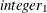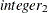… <options> ;

The SPLINESEASON statement is used to specify a seasonal pattern that is to be approximated by a given B-spline. If the specified spline has degree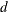and is based oninternal knots, then it can be written as a linear combination of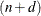regressors that are derived from the seasonal dummy regressors. The SPLINESEASON specification is equivalent to specifying a RANDOMREG specification with these derived regressors. Such approximation is useful only if the season length is relatively large, at least larger than. For additional information about splines, see Chapter 97: The TRANSREG Procedure in SAS/STAT 12.1 User's Guide,. For an example of using this statement, see Example 35.3.

DEGREE=integer

specifies the degree of the spline. It can be any integer greater than or equal to zero. The default value is 3.

KNOTS=lists the internal knots. This list of values must be a nondecreasing sequence of integers within the range of 2 to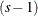, whereis the season length specified in the LENGTH= option. This is a required option in this statement.

LENGTH=integer

specifies the season length, s. This is a required option in this statement. The length can be any integer greater than or equal to three.

NOEST

fixes the value of the regression coefficient random walk disturbance variance to the value specified in the VARIANCE= option.

OFFSET=integer

specifies the position of the first measurement within the season, if the first measurement is not at the start of the season. The OFFSET= value must be between one and the season length. The default value is one. The first measurement refers to the start of the estimation span and the forecast span. If these spans differ, their starting measurements must be separated by an integer multiple of the season length.

PLOT=FILTER
PLOT=SMOOTH
PLOT=( <FILTER> <SMOOTH> )

requests plots of the season component. When you specify only one plot request, you can omit the parentheses around the plot request. You can use the FILTER and SMOOTH options to plot the filtered and smoothed estimates of the season component.

PRINT=FILTER
PRINT=SMOOTH
PRINT=( <FILTER> <SMOOTH> )

requests the printing of the filtered or smoothed estimate of the spline season component.

RKNOTS=(knot, …, knot ) …(knot, …, knot )

specifies a grouping of knots such that the knots within the same group have identical seasonal values. The knots specified in this option must already be present in the list specified by the KNOTS= option. The knot groups must be non-overlapping and without any repeated knots.

VARIANCE=value

specifies an initial value for the regression coefficient random walk disturbance variance during the parameter estimation process. Any nonnegative value, including zero, is an acceptable starting value.